Courses

# Previous Year Questions: Triangles Class 10 Notes | EduRev

## Class 10 : Previous Year Questions: Triangles Class 10 Notes | EduRev

The document Previous Year Questions: Triangles Class 10 Notes | EduRev is a part of the Class 10 Course Mathematics (Maths) Class 10.
All you need of Class 10 at this link: Class 10

Short Answer Type Question

Q.1.  In Fig., ABC is an isosceles triangle right angled at C with AC = 4 cm. Find the length of AB.    [CBSE Allahabad 2019]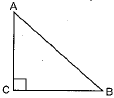Ans.
Given: AC = 4 cm
For isosceles triangle,
AC = BC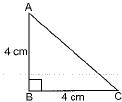∴ BC = 4 cm
Using Pythagoras theorem,
AB2 = AC2 + BC2
AB2 = (4)2 + (4)
⇒ AB2 = 16 + 16 = 32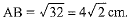Q.2. The area of two similar triangles is 25 sq. cm and 121 sq. cm. Find the ratio of their corresponding sides.    [CBSE 2019 (30/5/1)]
Ans.
The ratio of corresponding sides of two similar triangles.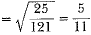The required ratio is 5: 11.

Q.3. In ΔABC, ∠B = 90° and D is the mid point of BC. Prove that AC2 = AD2 + 3CD2.    [GBST 2019 (30/5/1)]
Ans.
We have ΔABC is a right triangle right angled at B.
Also, D is the midpoint of BC.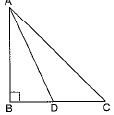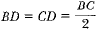In right ΔABC, we have
AC2 = AB2 + BC2
⇒ AC2 = AB2 + (2CD)2
⇒ AC2 = AB2 + 4CD2 ... (i)
In ΔABD, we have
AD2 = AB2 + BD2
⇒ AB2 = AD2 - BD2 = AD2 - CD2
Putting the value in (i), we have
AC2 = AD2 - CD2 + 4CD2 = AD2 + 3CD2
⇒ AC2 = AD2 + 3CD2 Hence proved.

Q.4. In the given Fig. 7.35, AB = AC. E is a point on CB produced. If AD is perpendicular to BC and EF perpendicular to AC, prove that ΔABD is similar to ΔECF.    [CBSE 2019 (30/5/1)]
Ans.
AB = AC (Given)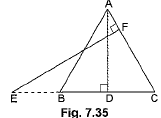⇒ ∠ABC = ∠ACB (Equal sides have equal opposite angles)
Now, in ΔABD and ΔECF
∠ABD = ∠ECF (Proved above)
∠ADB = ∠EEC (Each 90°)
So, ΔABD ∼ ΔECF (By AA similarity)

Q.5. Prove that the area of an equilateral triangle described on one side of the square is equal to half the area of the equilateral triangle described on one of its diagonal.    [CBSE 2018]
Ans.
Let the side of square ABCD be a units
Given: Let, the equilateral triangle formed on one side of the square is ΔAED and another equilateral triangle formed on its diagonal BD is ΔBDF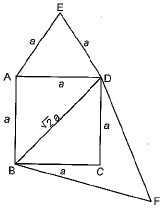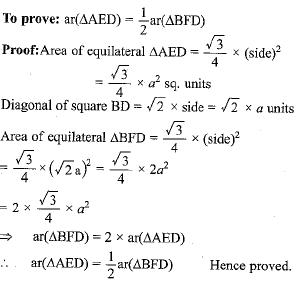Q.6. Given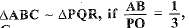then find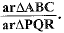[CBSE 2018]
Ans.
Since, ΔABC ∼ ΔPQR,Long Answer Type Question

Q.1. D and E are points on the sides CA and CB respectively of a triangle ABC right angled at C. Prove that AE2 + BD2 = AB2 + DE2.    [CBSE 2019]
Ans.
In right-angled ΔACE and ΔDCB, we have
AE2 = AC2 + CE2 (Pythagoras Theorem ) ...(i)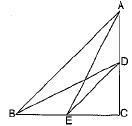and BD2 = DC2 + BC2 ...(ii)
Adding (i) and (ii), we have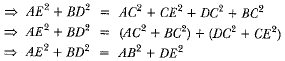(∵ AC2 + BC2 = AB2 and DC2 + EC2 = DE2 in right-angled triangle ABC and CDE respectively.)

Q.2. In a triangle, if the square on one side is equal to the sum of the squares on the other two sides, prove that the angle opposite to the first side is a right angle.    [CBSE 2019 (30/5/1)]
Use the above theorem to find the measure of ∠PKR.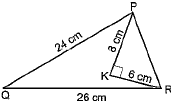Ans.
Given: A triangle ABC in which AC2 = AB2 + BC2.
To Prove: ∠B = 90°.
Construction: We construct a ΔPQR right-angled at Q such that PQ = AB and QR = BC
Proof: In ΔPQR, we have,
PR2 = PQ2 + QR2 (Pythagoras Theorem)
or, PR2 = AB2 + BC2 (By construction) ...(i)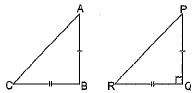But AC2 = AB2 + BC2 (Given) ...(ii)
So, AC2 = PR2 (From (i) and (ii))
∴ AC = PR ...(iii)
Now, in ΔABC and ΔPQR,
AB = PQ (By construction)
BC = QR (By construction)
AC = PR (Proved in (iii))
So, ΔABC ≌ ΔPQR (By SSS congruency)
Therefore,
∠B = ∠Q (CPCT)
But ∠Q = 90°  (By construction)
So, ∠B = 90°
Second part:
In ΔPQR,
By Pythagoras Theorem, we have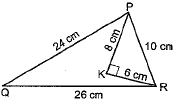PR2 = (26)2 - (24)2
⇒ PR2 = 676 - 576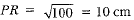Now, in ΔPKR we have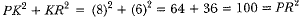Hence, ∠PKR = 90° (By Converse of Pythagoras Theorem)

Q.3. Prove that, in a right triangle, the square on the hypotenuse is equal to the sum of the squares on the other two sides.    [CBSE 2018]
Ans.
Given: ΔABC is a right-angled triangle, where ∠A = 90°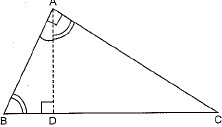To prove: BC2 = AB2 + AC2
Construction: Draw AD ⊥ BC
Proof: In ΔBDA and ΔBAC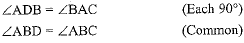∴ ΔBDA ~ ΔBAC (AA similarity)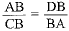(Corresponding sides of similar triangles)
⇒ AB2 = CB.DB ...(i)
Also, in ΔADC and ΔBAC,
∠ADC = ∠BAC (Each 90°)
∠ACD = ∠ACB (Common)
∴ ΔADC ~ ΔBAC (AA similarity)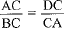(Corresponding sides of similar triangles)
⇒ AC2 = BC.DC ...(ii)
On adding (i) and (ii), we get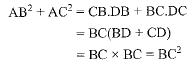∴ AB2 + AC2 = BC2 Hence proved

Offer running on EduRev: Apply code STAYHOME200 to get INR 200 off on our premium plan EduRev Infinity!

## Mathematics (Maths) Class 10

62 videos|363 docs|103 tests

,

,

,

,

,

,

,

,

,

,

,

,

,

,

,

,

,

,

,

,

,

;